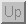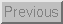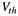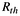Next: Signal Energy to Voltage Up: Additional Signal Conditioning Circuits Previous: Additional Signal Conditioning Circuits

### Comparators

Sometimes there is no need to send the entire range of voltages from a sensor to the analog-to-digital converter (ADC). Instead, many times a sensor is used simply as a switch. Figure 40 contains a circuit called a comparator which takes an analog sensor voltage and compares it to a threshold voltage,. If the sensor's voltage is greater than the threshold, the output of the circuit is maximum (typically 5V). If the sensor's output is less than the threshold, the output of the circuit is minimum (usually 0V). The threshold voltage is set by adjusting the potentiometer labeled. The output of the sensor can also be reduced by using the resistor divider network as shown if desired. Notice that the circuit has a positive feedback resistorwhich assures that the output of the comparator will swing quickly and completely from maximum output to minimum output (also called ``rail to rail'').

Example - Using an FSR as a Switch

An example of when this might be useful is in the case of the force sensing resistor. As depicted in Figure 6, typical force sensing resistors exhibit a region in which the resistance varies rapidly in response to a rather small variation in force. The force which defines this ‘knee' in the force vs. resistance curce is known as the break force. This behavior can be used to create a switch out of an FSR. This type of behaviour might be useful in devices such as membrane style keypads.Figure 40: An FSR in a Switch Configuration, Using a Comparator

Figure 40 shows a circuit which implements a switch based upon a force sensing resistor. The variable resistor,is used to set the sensitivity of the switch.

Tim Stilson
Thu Oct 17 11:25:23 PDT 1996